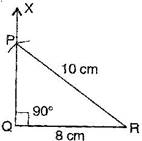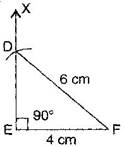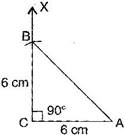### Practical Geometry - Solutions 5

CBSE Class –VII Mathematics
NCERT Solutions
Chapter 10 Practical Geometry (Ex. 10.5)

Question 1. Construct the right angled $\mathrm{\Delta }$PQR, where $m\mathrm{\angle }$Q = ${90}^{\circ },$ QR = 8 cm and PR = 10 cm.
Answer: To construct: A right angled triangle PQR where $m\mathrm{\angle }$Q = ${90}^{\circ },$ QR = 8 cm and PQ = 10 cm.Steps of construction:
(a) Draw a line segment QR = 8 cm.
(b) At point Q, draw QX $\mathrm{\perp }$ QR.
(c) Taking R as centre, draw an arc of radius 10 cm.
(d) This arc cuts QX at point P.
(e) Join PQ.
It is the required right angled triangle PQR.
Question 2. Construct a right angled triangle whose hypotenuse is 6 cm long and one the legs is 4 cm long.
Answer: To construct: A right angled triangle DEF where DF = 6 cm and EF = 4 cmSteps of construction:
(a) Draw a line segment EF = 4 cm.
(b) At point E, draw EX $\mathrm{\perp }$ EF.
(c) Taking F as centre and radius 6 cm, draw an arc. (Hypotenuse)
(d) This arc cuts the EX at point D.
(e) Join DF.
It is the required right angled triangle DEF.
Question 3. Construct an isosceles right angled triangle ABC, where $m\mathrm{\angle }$ACB = ${90}^{\circ }$ and AC = 6 cm.
Answer: To construct: An isosceles right angled triangle ABC where $m\mathrm{\angle }$C = ${90}^{\circ },$ AC = BC = 6 cm.Steps of construction:
(a) Draw a line segment AC = 6 cm.
(b) At point C, draw XC $\mathrm{\perp }$ CA.
(c) Taking C as centre and radius 6 cm, draw an arc.
(d) This arc cuts CX at point B.
(e) Join BA.
It is the required isosceles right angled triangle ABC.